# Slope

In past lessons we have already learnt many facts about the slope. It is essentially a measurement of the steepness of a line. In the equation $$y = m(x)+b$$, $$m$$ is the slope.

## Types of Slope (+/-, 0, undefined)

 A positive slope means that two variables are positively related; that is, when x increases, so does y, and when x decreases, y also decreases.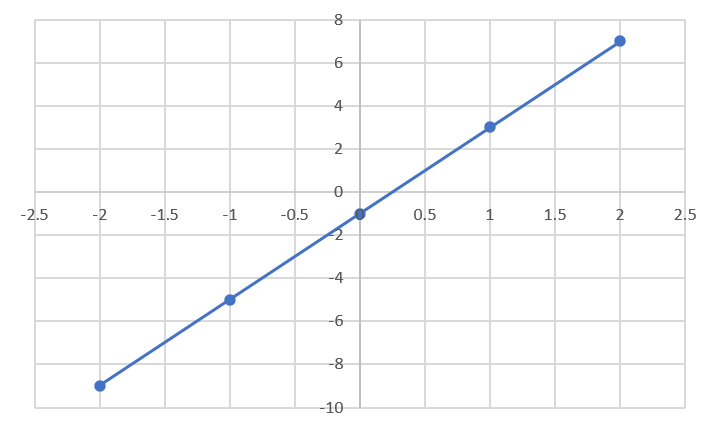A negative slope means that two variables are negatively related; that is, when x increases, y decreases, and when x decreases, y increases.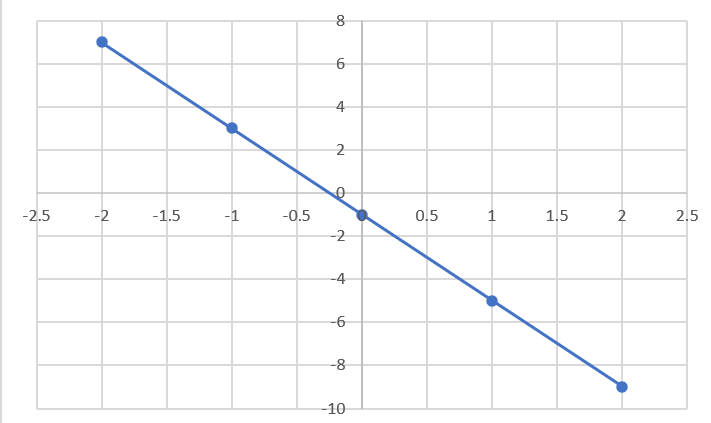A slope of zero is a horizontal line. That means that there is a constant relationship between x and y. The value of y does not change when x changes. These equations are written as $$y=b$$ where $$b$$ is the y-int.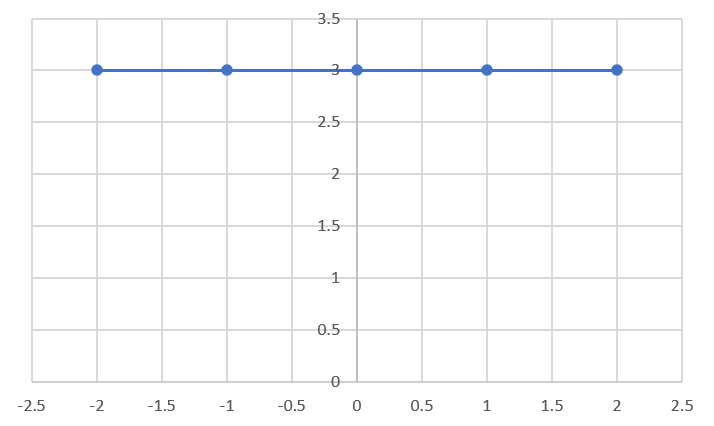An undefined slope (or an infinitely large slope) is the slope of a vertical line. The value of x does not change when y changes. These equation are usually written as $$x = a$$ where $$a$$ is the x-int.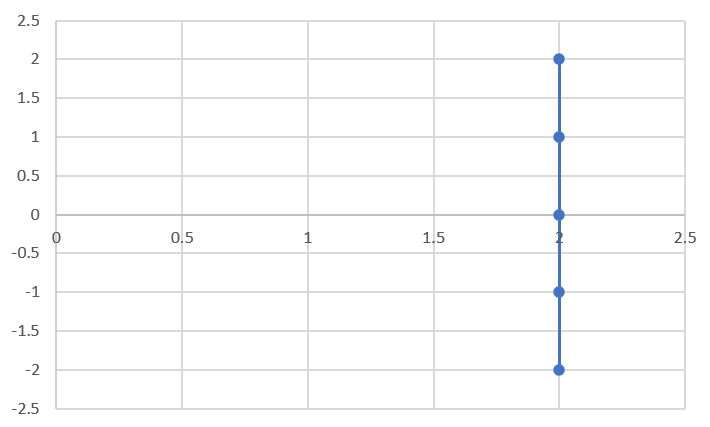## Slope Equation

To calculate the slope using two points on the line, we need to calculate the rise over the run. The rise is how much the line increases in the y-direction between two points. The run is the distance between the points in the x-direction between two points.

$$m = \cfrac{rise}{run} = \cfrac{\Delta y}{\Delta x} = \cfrac{y_2 - y_1}{x_2 - x_1}$$

This formula calculates the change in y (the rise) divided by the change in x (run) to measure the steepness (slope). First, select two points. Then plug in the x and y values into the equation to calculate the slope.

Let's try an example using the graph below. The first point is $$(x_1 = 1,y_1 = 3)$$. The second point is $$(x_2 = 2,y_2 = 7)$$. Now plug into the equation:

$$m = \cfrac{y_2 - y_1}{x_2 - x_1} = \cfrac{7 - 3}{2 - 1} = \cfrac{4}{1} = 4$$

The slope of the line is $$m = 4$$.

The slope of a line is constant meaning it doesn't matter which two points you pick, the slope will be the same! If we calcualte the slope using the points $$(x_1 = 1,y_1 = 3)$$ and $$(x_2 = 0,y_2 = -1)$$ we will get the same slope.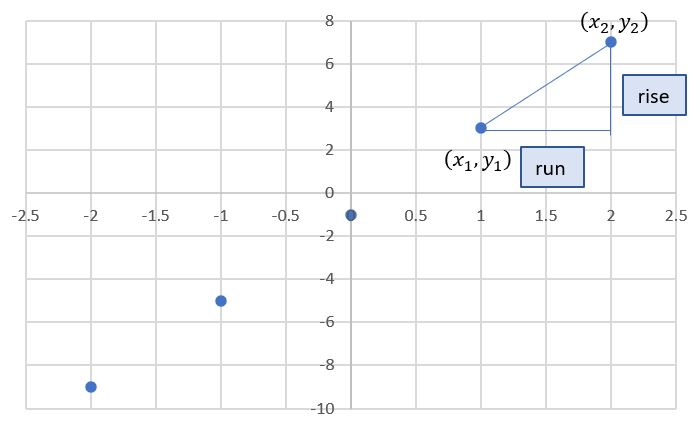Calculate the slope of the line from the table of values.
 X Y 2 4 6 8 10 12 11 10 9 8

## Parallel/Perpendicular Lines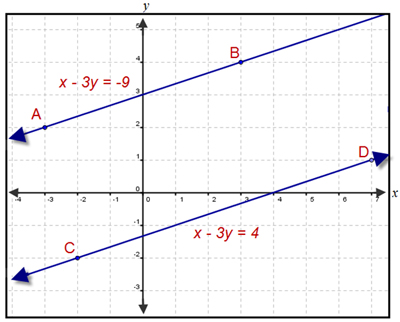Two lines are parallel if their slopes are equal and they have different y-intercepts. If the y-ints are the same, then they would be the same line!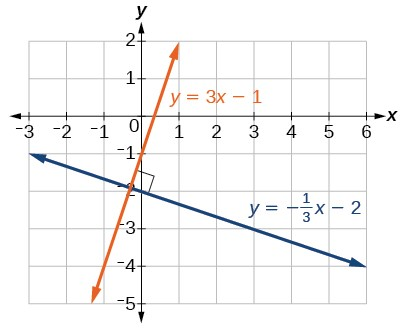Two lines are perpendicular if their slopes are negative reciprocals of each other. Remember, to find the reciprocal of a number, switch the numerator and denominator (flip the fraction).

Find the equation of any line that is a) parallel and b) perpendicular to the line: $$y = -7x + 5$$

Find the slope of the line that is a) parallel and b) perpendicular to the line: $$\cfrac{-2}{3}(x) + y = 0$$

Write an equation for the line in slope/intercept form that passes through (-2, -5) and parallel to the line: $$y = 4x - 1$$.